### Turbulent Flow in Open Channels

The turbulent flow in open channels can be approximated by one-dimensional network calculations. For the theoretical background the reader is referred to  and expecially  (in Dutch). The governing equation is the Bresse equation, which is a special form of the Bernoulli equation: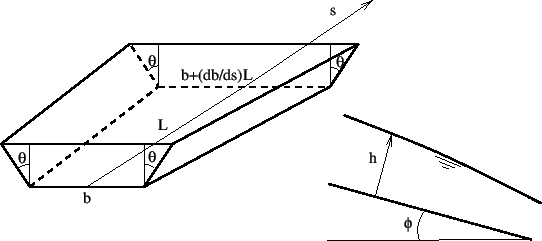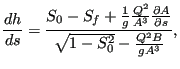(413)

where (Figure 137) h is the water depth (measured perpendicular to the channel floor), s is the length along the bottom,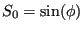, where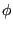is the angle the channel floor makes with a horizontal line,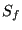is a friction term,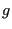is the earth acceleration,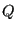is the volumetric flow (mass flow divided by the fluid density),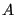is the area of the cross section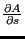is the change of the cross section with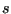keeping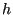fixed and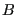is the width of the channel at the fluid surface. The assumptions used to derive the Bresse equation are:

2. each cross section is hydrostatic
3. the velocity is constant across each cross section
4. the velocity vector is perpendicular to each cross section.

Forseveral formulas have been proposed. In CalculiX the White-Colebrook and the Manning formula are implemented. The White-Colebrook formula reads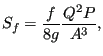(414)

where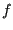is the friction coefficient determined by Equation 150, and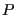is the wetted circumference of the cross section. The Manning form reads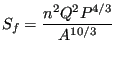(415)

where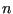is the Manning coefficient, which has to be determined experimentally.

In CalculiX the channel cross section has to be trapezoidal (Figure 137). For this geometry the following relations apply: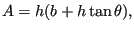(416)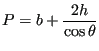(417)

and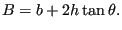(418)

Within an element the floor width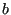is allowed to change in a linear way. All other geometry parameters are invariable. Consequently: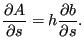(419)

The elements used in CalculiX for one-dimensional channel networks are regular network elements, in which the unknowns are the fluid depth and the temperature at the end nodes and the mass flow in the middle nodes. The equations at our disposal are the Bresse equation in the middle nodes (conservation of momentum), and the mass and energy conservation (Equations 407 and 411, respectively) at the end nodes.

Channel flow can be supercritical or subcritical. For supercritical flow the velocity exceeds the propagation speed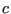of a wave, which satisfies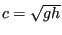. Defining the Froude number by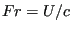, where U is the velocity of the fluid, supercritical flow corresponds to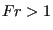. Supercritical flow is controlled by upstream boundary conditions. If the flow is subcritical (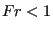) it is controlled by downstream boundary conditions. In a subcritical flow disturbances propagate upstream and downstream, in a supercritical flow they propagation downstream only. A transition from supercritical to subcritical flow is called a hydraulic jump, a transition from subcritical to supercritical flow is a fall. At a jump the following equation is satisfied  (conservation of momentum):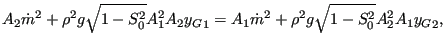(420)

where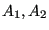are the cross sections before and after the jump,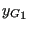and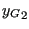are the centers of gravity of these sections,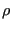is the fluid density and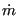is the mass flow. A fall can only occur at discontinuities in the channel geometry, e.g. at a discontinuous increase of the channel floor slope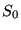. Available boundary conditions are the sluice gate, the weir and the infinite reservoir. They are described in Section 6.6.

Output variables are the mass flow (key MF on the *NODE PRINT or *NODE FILE card), the fluid depth (key PN -- network pressure -- on the *NODE PRINT card and DEPT on the *NODE FILE card) and the total temperature (key NT on the *NODE PRINT card and TT on the *NODE FILE card). These are the primary variables in the network. Internally, in network nodes, components one to three of the structural displacement field are used for the mass flow, the fluid depth and the critical depth, respectively. So their output can also be obtained by requesting U on the *NODE PRINT card. This is the only way to get the critical depth in the .dat file. In the .frd file the critical depth can be obtained by selecting HCRI on the *NODE FILE card. Notice that for liquids the total temperature virtually coincides with the static temperature (cf. previous section; recall that the wave speed in a channel with water depth 1 m is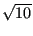m/s). If a jump occurs in the network, this is reported on the screen listing the element in which the jump takes place and its relative location within the element.